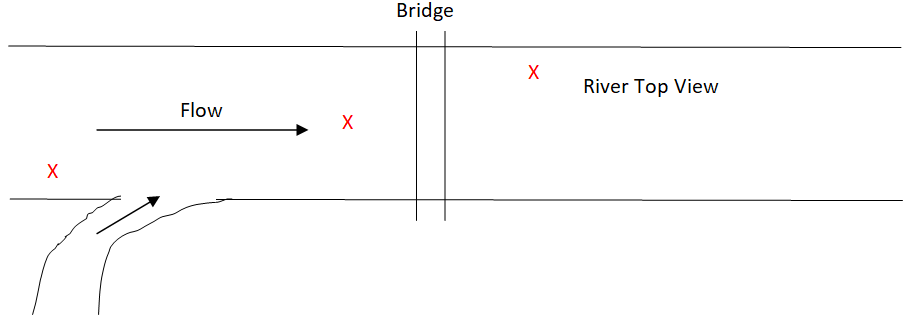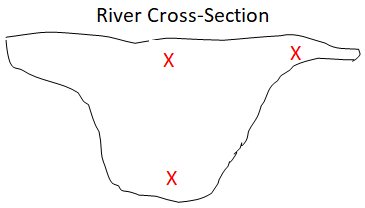# Development of a Sampling Plan

1) Quickly, in ~1-2 minutes, write down three things that you would consider to be the most important when sampling.

1. Where/when do we collect samples? Why does it matter where/when we collect our samples? (Random vs. Judgemental vs. Systematic, Convenience, or combinations of these)

Because our samples are always going to have some level of heterogeneity. If we collect one sample from one location, can we be certain that the value is representative of the target population?

In the two different sampling scenarios below (marked by X’s), discuss a disadvantage and advantage to sampling in these locations, followed by phosphorus detection?Drawing a sketch of three rivers below, place X’s on the three rivers to describe random, judgmental, and systematic sampling.

2. What kind of sample do we collect? Describe which type(s) of sample (Grab vs. Composite vs. In-Situ) is appropriate in the following situations:

-If we are determining the blood glucose level in a diabetic patient

-In a soil sample to determine average iron content

-In a 100-ft deep lake

-In a wastewater treatment plant required to report the average concentration of free ammonia discharged into their retention basin.

3. How much sample do we collect? What practical factors can lead to our decision here?

What statistical factors limit us here?

4. How do we limit uncertainty in our analysis? Consider the following flow chart leading to sample analysis.-When we sample, prepare a sample for analysis, and perform the analysis, there is a level of uncertainty in our measurement. How can we quantify the overall uncertainty?

-What is the best way to decrease the overall uncertainty?

You have determined the following variances for each step in the procedure:

S2(samp) = 3.3 x 10-3

S2(prep) = 1.2 x 10-4

S2(analysis) = 1.5 x 10-7

What part of our procedure should we focus on to improve the overall uncertainty (i.e. sampling, sample prep, analysis)?